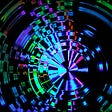# Who (and where) are we in this world?

We, humans, tend to have quite the memory. Especially when driving. We know what a red traffic light is and realize that we need to deaccelerate our cars, remember road names and even perform path planning with Google Maps, to even understand how to drive a car!

Let’s say I give you this image of the world map and ask you to pinpoint (to the nearest city) of where I am. You probably have no idea. I could be in San Francisco all the way to Sydney or even Buenos Aires.

But let’s say I give you this image below.

Mathematically speaking, the Gaussian distribution of the initial prediction I asked you to make (when I asked you to tell me where I am by simply giving the world map) is completely even.

Let me give you one more image and now let’s see if you can figure out where I am:

When I gave you a specific image, the Effiel Tower, you were able to pinpoint the exact city I was talking about given that data point. You’ve figured out the mean of the Gaussian ( μ) with very low variance (σ).

This is essentially the heart of localization in autonomous vehicles. Given an initial GPS estimate of our location, we can use landmarks as a source to help localize and figure out where we (the self-driving car) are in the world.

# GPS sucks

A lot of people generally use GPS to drive when on highways, that’s fine. But in the case of autonomous vehicles, GPS signals tend to have a variance (σ) of between 3 and 10 meters which is extremely inaccurate. The GPS might be telling me that I’m driving in the right lane but in reality, I’m completely off track and probably hit a few pedestrians.

GPS is a great place to start with in terms of localizing a self-driving car. The problem though is simply the fact that it’s an inaccurate guess to believe that GPS is the solution to localization.

GPS helps autonomous vehicles with a rough estimate as to where the car is (in math terms, that’d be a Gaussian with 0 means). Initially, the car could literally be anywhere in the world. But GPS allows the car to get a better, updated estimation as to where it is. The mean would be higher than what it would be before (because we’ve pinpointed the location) with a drastic decrease of variation (from any coordinate point in the whole world to coordinate points between 3–10meters).

In order to build a better estimation system, we’re going to have to start at our beautiful Kalman Filters.

# Using Sensor Fusion data

The first step with localizing autonomous vehicles comes from gathering our data from Sensor Fusion. I wrote an article going more in-depth about this which you can read here. (Note: this section assumes that you’ve read the article that I’ve linked on Sensor Fusion or you know how Kalman Filters + Sensor Fusion works).

When we take in Sensor Fusion data, we essentially get a list of all the objects that are detected through the LiDAR and Radar sensors of the car. We also get the coordinates of landmarks (ex. buildings) that don’t “move” over time to help figure out where we might be.

What’s really interesting is that we can use a nearest-neighbours algorithm to actually help us figure out where the car might be. For every single point in the Sensor Fusion data, the nearest-neighbour algorithm will associate the closest point to each landmark. Based on that, we’re able to figure out where we can potentially be.

The problem is simply the fact that this is not an extremely accurate method and we’ll need something even more accurate to make predictions. But, let’s use this as an initial estimate as to where we could be, on top of our GPS estimates.

# Particle filters

The GIST of Particle filters is that they perform the realization of Bayes Rule. We scatter a bunch of points of all the possibilities we could be at (Gaussian with 0 mean) and associate each point with a weight of 3 dimensions [x, y, θ] where x and y are our coordinate points and θ is our heading.

What you notice in this image is that all the red dots placed around the whole map are all the possibilities where the car might be. The blue lines are landmark locations from Sensor Fusion data (pinpointing how far each landmark is from us) with the nearest neighbour implemented in order to better understand our distance from the landmark. Each particle has a weight associated with it which we can deem as important weights. The more important the weight, the higher probability it will survive (weight size is directly proportional as to how long the estimate will last).

This is exactly shown in the GIF below. The weights are resampled at every single timestep and the bigger the weight is, the higher probability that it actually survives.

This can be broken down into 4 main steps:

1. Initialization
2. Prediction step
3. Update step (weights)
4. Resampling

# Initialization

The first step with Initialization is to figure out how many particles (the “red dots”) we want to use for estimations (yup, this is a hyperparameter that we’d tune). You need to figure out the optimal value to use between accuracy and cost (computation cost → can make the car too slow).

The easiest way to initialize a car is simply to divide up the map/initial GPS estimate into evenly spaced grid sections while taking into account Gaussian sensor noise distribution.

# Prediction

Now that we’ve initialized our map space and initialized all the sensor data which can be passed into the prediction step, it’s time to do exactly that; make a prediction for every single particle as to where we might be. We would update each particle using the velocity and yaw measurements with Gaussian sensor noise using a basic motion model.

The formula below helps us exactly with that:

Earlier above in the article, I mentioned that each particle comes with an associated x, y, and θ while accounting for the Gaussian sensor noise. We use this simple motion model (which is updated based on velocity) to help estimate where the car might be.

# Update step (weights)

Data association is the problem that we have to solve before updating our weights to help map up our LiDAR measurements with the map landmark. This is exactly where the nearest neighbour algorithm comes into play. We compare the magnitude of all the points and use that data to help figure out which LiDAR point is closest to the actual landmark itself. This nearest neighbour assumption model would play a role in terms of updating the weights of the particles.

In the update step, instead of using our feature measurements (LiDAR + Radar) to affect the predictions about the location of the car, we can use the measurements to update the weights of the particles.

The way we can update the weights of the particles is through the use of the Multivariate Gaussian Probability Density function for each measurement and combine the likelihood of all the measurements by taking their product. Remember that we assume each landmark observation is independent so we will simply take the product of all the likelihoods over all the measurements.

xᵢ is our x measurement at time i with yᵢ is our x measurement at time i. Σ would be our covariance matrix, consisting of the Gaussian noise for x and y.

1 major thing to note is that we’d need to transform the car’s measurements from its local coordinate system to the map coordinate system (xy system → map).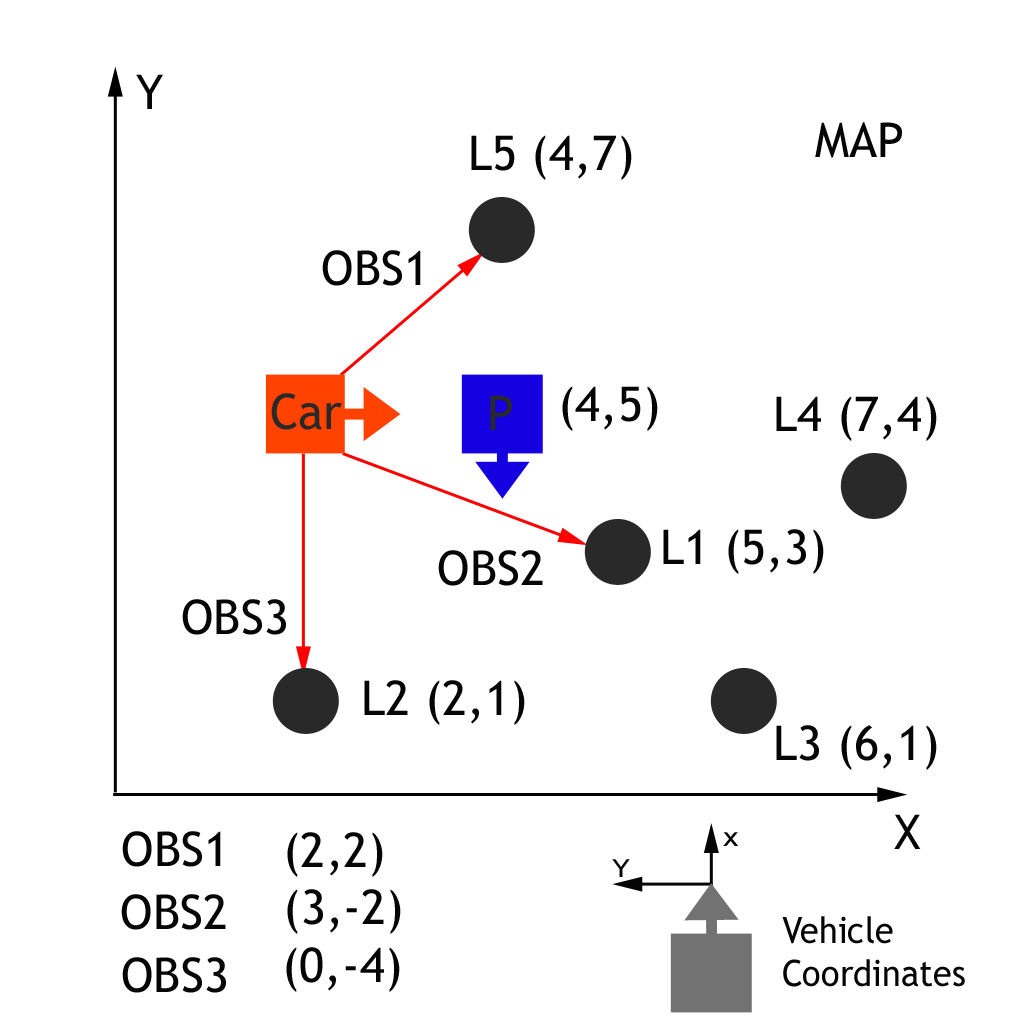Example of the car on the vehicle's coordinates. This needs to be transformed into the map’s coordinates. Source

The image above is an example of the car’s coordinates. We now want to get that into the coordinates of a map. This can be done using a Homogenous transformation using the equations below (here’s a great video to learn more about this transformation problem):

So to summarize, the update step consists of 3 parts:

1. Transformation from vehicle coordinates to map coordinates
2. Data association → use nearest neighbours algorithm to figure out which weights are closest to a landmark
3. Update step → use the Multivariate Gaussian Probability Density Function to update the weights of each particle

# Resampling

This is the real meat of localization; how do we get rid of the estimates and weights that don’t matter to us while keeping the estimates that help us localize the car?

Let’s say that we have a pie chart where we place all our weighted particles there (each particle takes the slice, the bigger the slice = bigger the importance weight %). What would happen is we’d define a variable, β (beta) that starts at 0. β’s value would then be updated by uniformly sampling a value between 0 and 2*the maximum weight (ex. if the biggest weight was 0.3, then the biggest weight would be 0.6).

We would also choose a random index, i for our weights. So, if we chose index 6 as an example (weight #6 on the pie chart), then we would compare the weight of that and β. If that weight is less than β, then we’d discard weight #6. Now, β is now interfering with weight #7 (the difference between β and weight #6 is what would move onto weight #7).

If β’s now updated value is less than weight #7, then we keep weight #7 and don’t discard it (it survives). Because now weight #7 is > β, we would now resample a new value for β and continue from there.

Sebastian Thrun explains this in-depth in his A.I for Robotics Class. Feel free to watch the video for more information. The visuals he provides are extremely helpful with developing the logical intuition behind the Resampling algorithm.

# Calculating the error

In terms of figuring out a method to measure the overall performance of our model, we can use the weighted average error of all the particles. This can be done by simply taking the root squared error between each particle and its ground truth and multiply it by the particle’s weight.

# Localization in action!

As part of the Become a Self-Driving Car Engineer nanodegree I’ve been doing, I’ve been tasked with this exact challenge below. Feel free to take a look at the Github code and the video demonstration below!

Note that the blue circle in the video is actually the estimate of the localized prediction of the car.

# Connect with me

Keep up with my progress and journey to build a fully self-driving car below!

Email

Website

--

--

--

## More from MLearning.ai

Data Scientists must think like an artist when finding a solution when creating a piece of code. ⚪️ Artists enjoy working on interesting problems, even if there is no obvious answer ⚪️ linktr.ee/mlearning 🔵 Follow to join our 18K+ Unique DAILY Readers 🟠

## Data Science Nano Degree Capstone Project: Using CNN to predict dog breed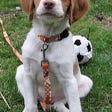## Reinforcement Learning Made Simple (Part 1): Intro to Basic Concepts and Terminology## SSD: Single Shot MultiBox Detector## An introduction to Machine Learning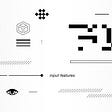## Single-layer Perceptron in Pharo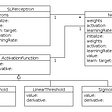## Reinforcement Learning Explained Visually (Part 4): Q Learning, step-by-step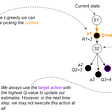## Machine learning LEGO image recognition: Using virtual data and YOLOv3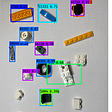## Sri Anumakonda

Building Self-Driving Cars as a 15yo. srianumakonda.com

## Living with Artificial Intelligence | Part 1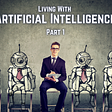## Self-supervised learning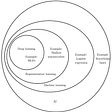## How a Neural Network is Trained?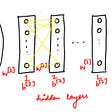## Quantum Computing in the Automotive Industry